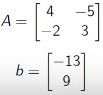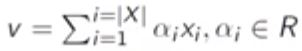# New Technology

Trending Technology Machine Learning, Artificial Intelligent, Block Chain, IoT, DevOps, Data Science

## Search This Blog

Linear Algebra :

Linear algebra is the branch of mathematics concerning vector spaces and linear mappings between such spaces. It includes the study of lines, planes, and subspaces, but is also concerned with properties common to all vector spaces.

Why do we study Linear Algebra ?
• Provides a way to compactly represent & operate on sets of linear equations.
• In machine learning, we represent data as matrices and hence it is natural to use notions and formalisms developed in Linear Algebra.
Consider the following system of equations :

4x1 - 5x2 = -13
-2x1 + 3x2 = 9

In matrix notation, the system is more compactly represented as :

Ax = bVector Space

A set V with two operations + and . is said to be a vector space if it is closed under both these operations and satisfies the following eight axioms.

Commutative Law

x + y  = y + x,     ∀x, y ∈ ⋁

Associative Law

(x + y) + z  =  x + (y + z),    ∀x, y, z ∈ ⋁

∃0 ∈ ⋁   s.t    x + 0  = x,      ∀x ∈ ⋁

∀x ∈ ⋁,     ∃X~    s.t  X + X~  =  0

Distributive Law

α . (x + y)  =  α . x + α . y,   ∀α ∈ R, x, y ∈ ⋁

Distributive Law

x . (α + β)  =  α . x + β . y,   ∀α,,β ∈ R, x ∈ ⋁

Associative Law

(α β) . x  = α . (β . x),    ∀α,,β ∈ R, x ∈ ⋁

Unitary Law

1 . x  = x.        ∀x ∈ ⋁

Subspace :

Let W be a subset of a vector space V. Then W is called a subspace of V if W is a vector space.
•  Do we have to verify all 8 condition whether a given subset of a vector space is a subspace ?
• Theorem : Let W be a subset space V. Then W is a subspace of V if and only if W is non-empty and      x + αy  ∈ W,    ∀x,y ∈ W, α ∈ R
Norm

The span of a set of vectors X = {x1, x2, ....xn} is the set of all vectors that can be expresses as a linear combination of the vectors in X.
In other words, set of all vector v such thatThe range or columnspace of a matrix A, denoted by R(A) is the span of its coulmns. In other words, it contains all linear combinations of the columns of A. For instance, the columnspace of

Nullspace Of A Matrix

The nullspace N(A) of a matrix A ∈ R{mxn)  is the set of all vectors that equal 0 when multiplied by A. The dimensionality of the nullspace is also referred to as the nullity of A.
Note that vectors in N(A) are of dimension n, while those in R(A) are of size m, so vector in R(At) and N(A) are both of dimension n.Another Example

Now, consider the matrix

Here, the third column is linear combination of the first two columns.
Here, the nullspace is the line of all points x = c, y = c,  z = -c.

Linear Independence and Rank

A set of vectors {x1, x2, ....xn) ∈ R power n is said to be (linearly) independent if no vector can be represented as a linear combination of the remaining vectors.

Properties of RankOrthogonal Matrices

A square matrix U ∈ R  is orthogonal ifff
• All columns are mutually orthogonal
If U is Orthogonal, UUT = UTU = 1 . This also implies that the inverse of U happens to be its transpose.

Another salient property of orthogonal matrices is that they do not change the Euclidean norm of a vector when they operate on it. i.e ||Ux||2 = ||x||2.
Multiplication by an orthogonal matrix can be thought of as a pure rotation, i.e., it does not change the magnitude of the vector , but changes the direction.